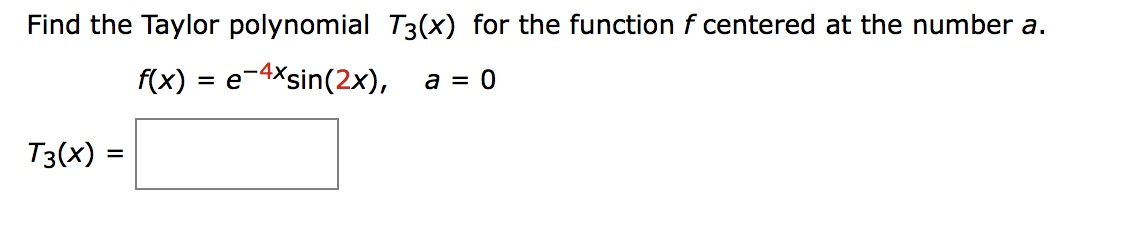Find the Taylor polynomial T3(x) for the function f centered at the number a.에rx) = e-Mein(2x),a = 0T3(x) =

Questionhelp_outlineImage TranscriptioncloseFind the Taylor polynomial T3(x) for the function f centered at the number a. 에 rx) = e-Mein(2x), a = 0 T3(x) = fullscreen
Step 1

The function is

Step 2

The formula to find the Taylor polynomial for the function f centered at the number a, is

Step 3

For n = 3...

Want to see the full answer?

See Solution

Want to see this answer and more?

Our solutions are written by experts, many with advanced degrees, and available 24/7

See Solution
Tagged in

Derivative